Latest Banking jobs   »   Quantitative Aptitude Quiz For RRB PO...   »   Quantitative Aptitude Quiz For RRB PO...

# Quantitative Aptitude Quiz For RRB PO Mains 2021- 13th September

Directions (1-5): The following questions are accompanied by two statements (I) and (II). You have to determine which statements(s) is/are sufficient/necessary to answer the questions.
(a) Statement (I) alone is sufficient to answer the question but statement (II) alone is not sufficient to answer the questions.
(b) Statement (II) alone is sufficient to answer the question but statement (I) alone is not sufficient to answer the question.
(c) Both the statements taken together are necessary to answer the questions, but neither of the statements alone is sufficient to answer the question.
(d) Either statement (I) or statement (II) by itself is sufficient to answer the question.
(e) Statements (I) and (II) taken together are not sufficient to answer the question.

Q1. Ratio between length of two trains is 4 : 3. What will be difference between lengths of both trains?
I . Speed of larger trains and smaller train is 72 km/hr and 90 km/hr respectively. Both trains cross each other in   28/3 sec, when running in opposite direction.
II . Speed of smaller train is 90 km/hr and it can cross a pole in 7.2 sec.

Q2. There are three men P, Q and R. Find the difference between time taken by P & Q together to complete a task and time taken by Q & R together to complete the same task?
I . ‘R’ takes twice as much time as ‘Q’ and thrice as much time as ‘P’ takes alone.
II . If they all three works together work will be completed in 4 days.

Q3. Ratio between length and breadth of rectangle ‘X’ is 7 : 4. Find area of a square ‘Y’?
I . length of rectangle ‘X’ is two times of radius of circle, whose area is 616 cm2.
II . Perimeter of rectangle ‘X’ is 20 cm more than perimeter of square ‘Y’.

Q4. Kumud’s mark in Geography are 20 more than the average marks obtained by him in English, History, Geography and Mathematics. What is the difference between the marks obtained by him in History and Mathematics?
I. Total marks obtained by him in Geography and History is 160
II. The total marks obtained by him in History, Geography and Mathematics is 210.

Q5. What is the minimum passing percentage in a test?
I. Raman scored 25% marks in the test and Sunil scored 288 marks which is 128 more than that of Raman.
II. Raman scored 64 marks less than the minimum passing marks.

Directions (6-10): The following questions are accompanied by two statements (I) and (II). You have to determine which statements(s) is/are sufficient/necessary to answer the questions.

Q6.C can finish a work in 10 days. Find the time taken by A and B together to finish the same work.
(i) Time taken by A, B and C together to complete the work is 4 days.
(ii) When they all start doing same work at same time, B will do 32% work till the time work is completed.
(a) Only I is required
(b) Only II is required
(c) Both I and II is required
(d) Either I or II is required
(e) Cannot be answered even from both I and II

Q7.Find overall loss or profit% of Ram if he bought 100 balls at the rate of 10 balls for x Rs. and sold them at the rate of y balls for 10 Rs.
(i) H.C.F. of x and y is 2.
(ii) Ratio of x to y is 5 : 4.
(a) Only I is required
(b) Only II is required
(c) Both I and II is required
(d) Either I or II is required
(e) Cannot be answered even from both I and II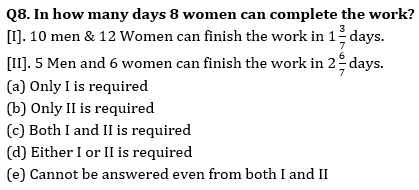Q9. What is the radius of the circle?
[I]. Length of a rectangle is 10% more than the breadth. Area of rectangle is 440 cm². Length of rectangle equals to circumference of circle.
[II] .Circumference of circle is equal to side of square having area 484 cm²
(a) Only I is required
(b) Only II is required
(c) Both I and II is required
(d) Either I or II is required
(e) Cannot be answered even from both I and II

Q10. Ram sold an item. Find the M.P of the item?
[I]. Ram gave two successive discounts is 20% & 5% on marked price. But after that take 25% more on discounted price as tax. Ram can earn 40 more If he sell the item at MP.
[II] .Ram gave two successive discount of MP i.e., 15% & 20% whereas Ram kept M.P. 50% more than the C.P. of that item.
(a) Only I is required
(b) Only II is required
(c) Both I and II is required
(d) Either I or II is required
(e) Cannot be answered even from both I and II

Solutions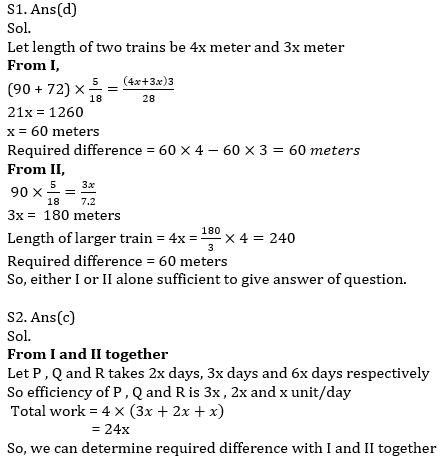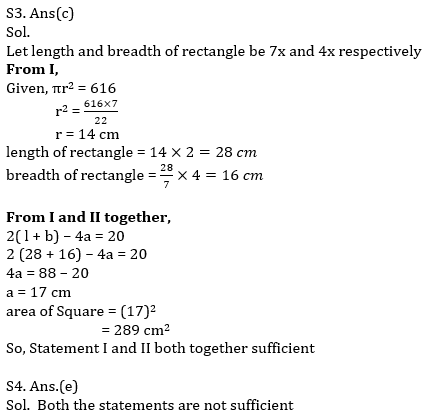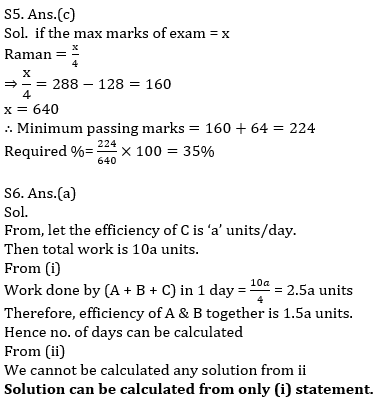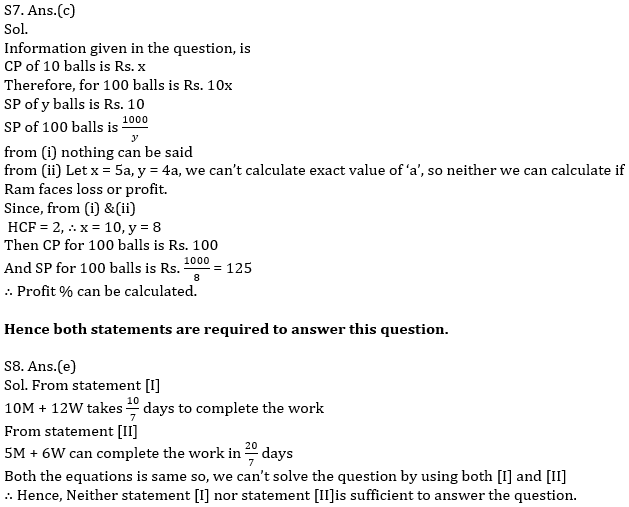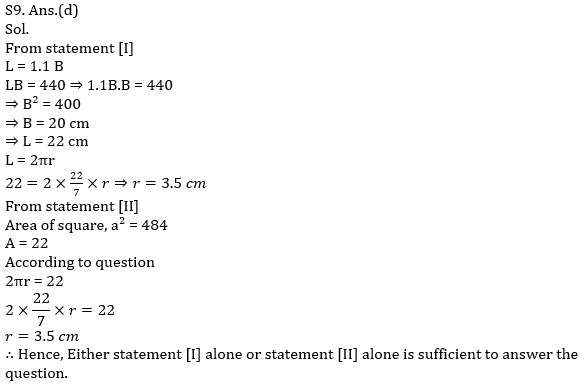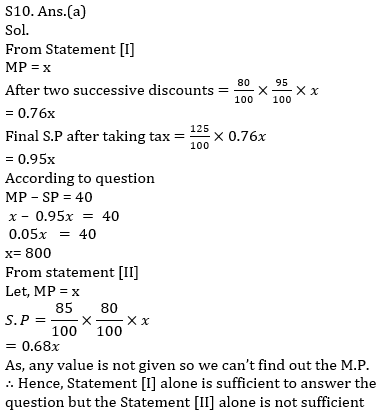Click Here to Register for Bank Exams 2021 Preparation Material#### Congratulations!Download Hindu Review of October 2021: Free PDF